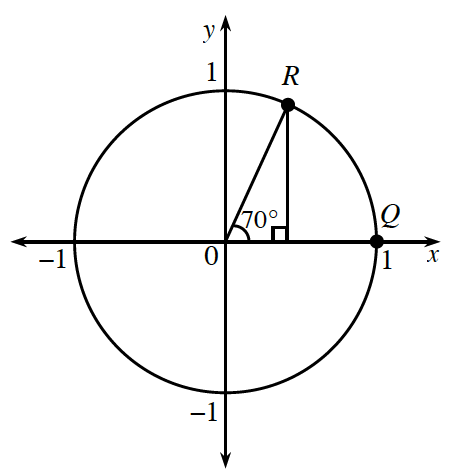### Home > CCA2 > Chapter Ch7 > Lesson 7.1.4 > Problem7-64

7-64.

A $70^\circ$ angle is drawn for you in the unit circle below.1. Approximate the coordinates of point $R$.

$\left(0.3420, 0.9397\right)$

2. How could you represent the exact coordinates of point $R$?

$\left(\cos70°, \sin70°\right)$

3. Show that the Pythagorean Identity works for this angle.

$\sin^\circθ \cos^\circθ = 1$